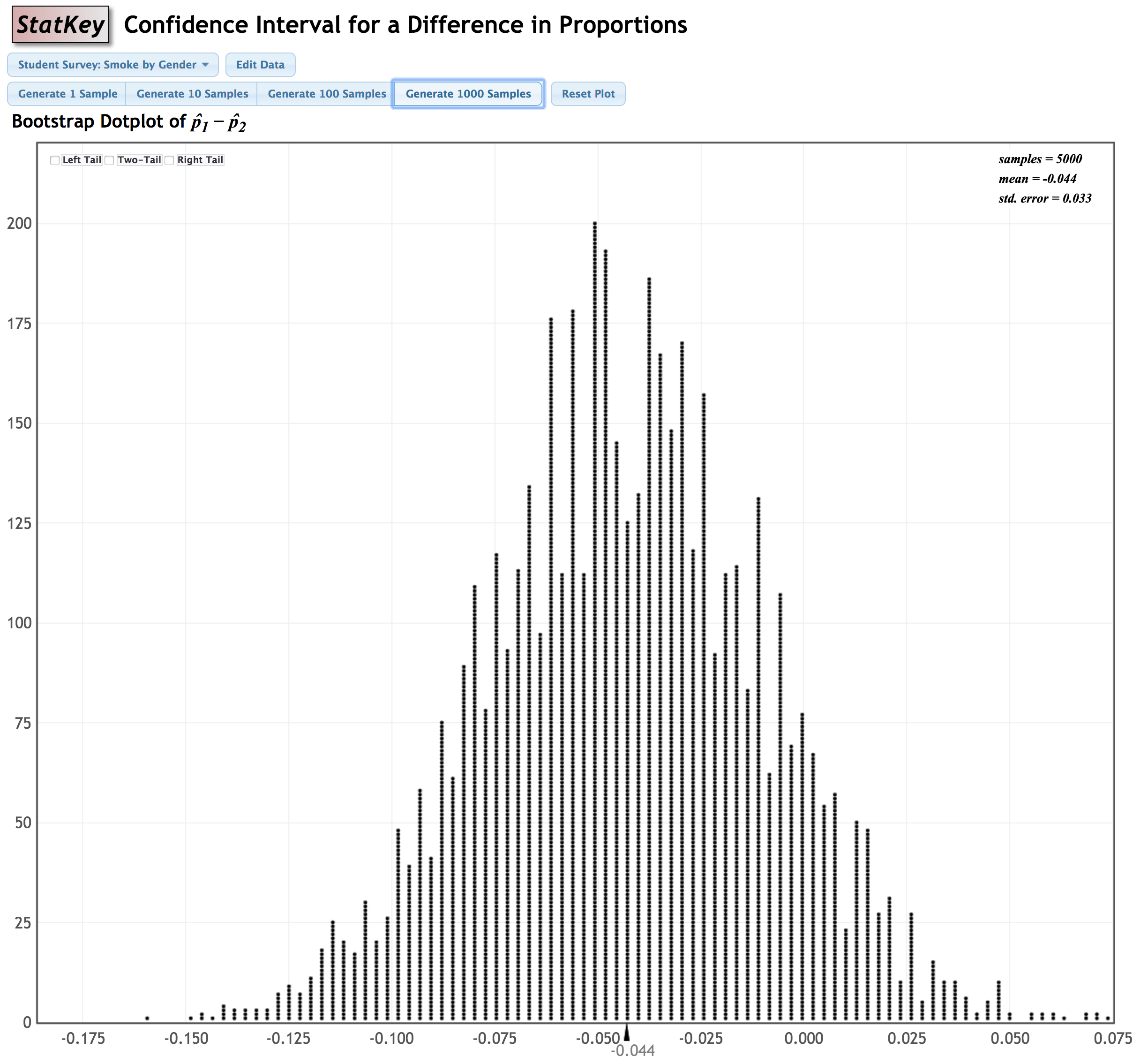# 7.4.2.4 - Example: 95% CI for Difference in Proportion of Smokers by Sex

Construct a 95% confidence interval to estimate the difference between the proportion of all females who smoke and the proportion of all males who smoke.

This dataset is built in to StatKey: Confidence Interval for Difference in Proportions. It is the Student Survey: Smoke by Gender dataset.

#### Original Sample

Group Count Sample Size Proportion
Female 16 169 0.095
Male 27 193 0.140
Female-Male -11 n/a -0.045

StatKey was used to construct a bootstrap sampling distribution:Because this distribution is approximately normal, we can approximate the sampling distribution using the z distribution. We will use the standard error, 0.033, from this distribution.

The original sample statistic was $$\widehat p_f - \widehat p_m = \frac{16}{169} - \frac{27}{193} = -0.045$$

We can find the $$z^*$$ multiplier for a 95% confidence interval using Minitab Express. This will be the values on a z distribution that separate the middle 95% from the outer 5%. (Note: You could apply the Empirical Rule and use a multiplier of 2, but the value found using Minitab Express will be more precise)The $$z^*$$ multiplier is 1.95996.

Recall the general form of a confidence interval: sample statistic $$\pm$$ $$z^*$$ (standard error) where $$z^*$$ is the multiplier. So in this case we have...

$$-0.045 \pm 1.95996(0.033)$$

$$-0.045 \pm 0.065$$

$$[-0.110,0.020]$$

I am 95% confident that the difference in the population between the proportion of females who smoke and the proportion of males who smoke (i.e., $$p_f-p_m$$) is between -0.110 and 0.020.